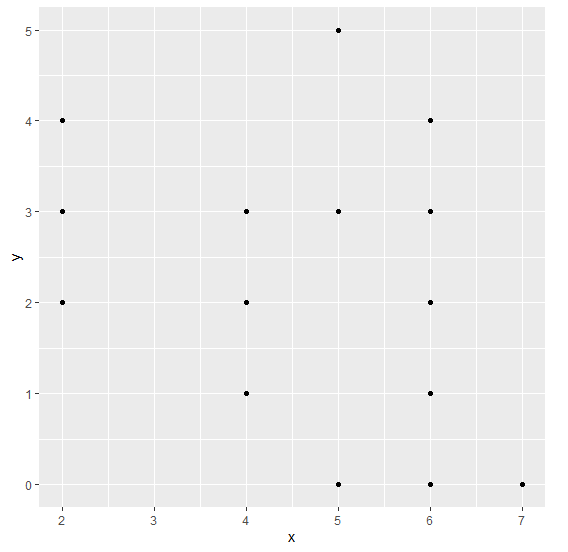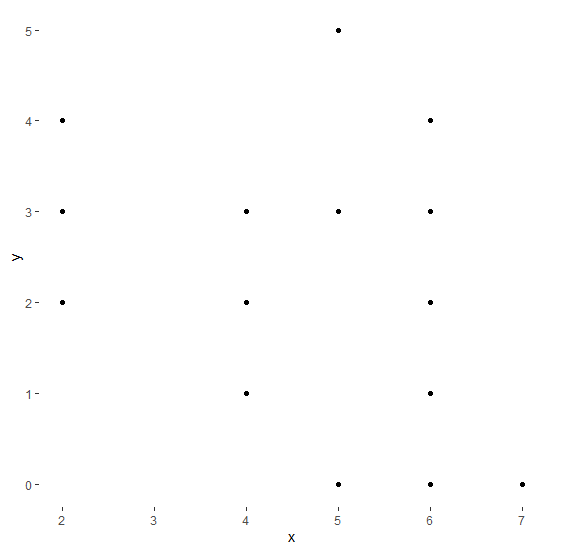# Create a graph without background panel using ggplot2 in R.

To create a graph without background panel, we can use theme function of ggplot2 package where we can set panel.background argument to blank.

For Example, if we have a data frame called df that contains two columns say x and y then we can create scatterplot between x and y without background panel using ggplot2 by using the below command −

ggplot(df,aes(x,y))+geom_point()+theme(panel.background=element_blank())

## Example

Consider the below data frame −

x<-rpois(20,5)
y<-rpois(20,2)
df<-data.frame(x,y)
df

The following dataframe is created

   x y
1 7 0
2 4 1
3 4 3
4 2 4
5 7 0
6 6 1
7 6 1
8 6 4
9 6 0
10 4 3
11 2 2
12 6 2
13 4 1
14 4 3
15 4 2
16 2 3
17 5 0
18 5 3
19 5 5
20 6 3

To load the ggplot2 package and create scatterplot between x and y on the above created data frame, add the following code to the above snippet −

x<-rpois(20,5)
y<-rpois(20,2)
df<-data.frame(x,y)
library(ggplot2)
ggplot(df,aes(x,y))+geom_point()

## Output

If you execute all the above given snippets as a single program, it generates the following Output −To create scatterplot between x and y without background panel on the above created data frame, add the following code to the above snippet −

x<-rpois(20,5)
y<-rpois(20,2)
df<-data.frame(x,y)
library(ggplot2)
ggplot(df,aes(x,y))+geom_point()+theme(panel.background=element_blank())

## Output

If you execute all the above given snippets as a single program, it generates the following Output −